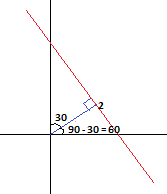mycollegehive
Find the equation of the straight line whose perpendicular f...
math0Find the equation of the straight line whose perpendicular from the origin is of length 2 units and is at an angel of 30to the positive x-axis

A. y+root3x=4

B. y-root3x=4

C. 4y+root3x=4

D. None of the above

177 viewsShareFollowNigeria
01 October 2020University of Benin Nigeria
07 November 20200The equation of a straight line is given by:

y = mx + c

where m is the slope and c is the intercept

let m1 be the slope of the perpendicular line and m2 be the slope of the straight line

m1 = tan(60) = √3

since m1 and m2 are perpendicular, m2 = -1/m1

m2 = -1/√3

Thus, y = -1/√3 x + c

multiply through by √3

y√3 = - x + c√3

x + y√3 - c√3 = 0 ------------- eqn(1)

d = |(ax1 + by1 + c)/(√a2 + b2)|

x1, y1 = 0

d = distance from the origin to the perpendicular length = 2

from eqn(1),

a = 1 [coefficient of x],

b = √3 [coefficient of y]

c = - c√3 [constant]

2 = |(c)/(√a2 + b2)|

2 = |(-c√3)/(√12 + √32)|

2 = |(-c√3)/(√1 + 3)|

2 = |(-c√3)/(√4)|

2 = |(-c√3)/2|

2 = (c√3)/2

c√3 = 4

substitute into eqn(1),

x + y√3 - 4 = 0

x + √3 y = 4Share

### Groups

How to insert math formulas/equations### Related Tags

math

23 followers

1262 questions